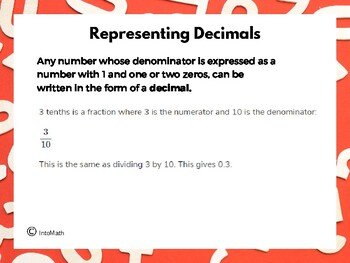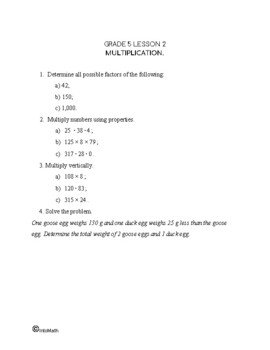CA$2.00 In this lesson you will learn about decimals and their properties. A decimal number can be defined as a number whose whole and part are separated by a decimal point. The digits following the decimal point show a value less than one. Decimals are based on the powers of 10. As we move from left to right, the place value of the subsequent digit is divided by 10, meaning the place value becomes tenths, hundredths and thousandths. One tenth is 1/10. In decimal form, it is 0.1. This concept is frequently used in everyday life and can represent many different quantities, relationships and situations.Grade 5 Workbook (16 pages with answers) CA$4.00

We have already seen how it can be used to represent dollars and cents.

We use decimals when we measure something. For example, when we step on a digital scale, it shows our exact weight as a decimal. Or maybe we want to measure the length, width or height of an object. Decimals are used to state the exact dimensions. We use the concept of decimals when filling up the gas or when determining the discount at a store. We also use them when we cook according to a recipe or when we are timing using a stopwatch.

You will also learn and practice how to identify the place value of each digit within a decimal number, as well as how to add and subtract decimals.

You will discover how to round decimals and why some of them are finite, while others can go on indefinitely.

For example, a Pi number goes on forever and mathematicians like to compete in reciting the digits of the number. The Pi number is a very special decimal number and is used in a variety of situations (when working with the radius and the diameter of a circle).